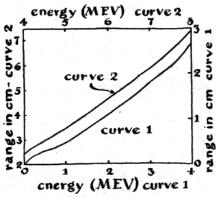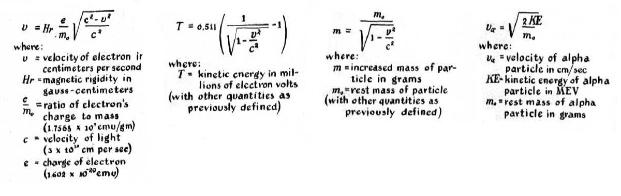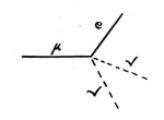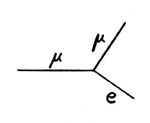Cloud Chambers
Ron Fleshman  KB2VIV
All cloud chambers work the same way.  As particles pass through a gas at high speed, their interactions with the
gas atoms produce ions.  If this area is supersaturated with a liquid vapor, and the temperature is below the dew
point, droplets of water quickly form on the ions.  A speeding particle leaves thousands of ions in its wake; the
resulting droplets comprise the vapor trail seen in the cloud chamber.  These trails show the path of the particle.  By
studying these trails or photographs of these trails, we can learn many things about the particles that produced them.
Alpha particles produce a thick heavy track.  This is due to the heavy ionization caused by the alpha particles large
mass (~8000 times that of an electron).  We can find the kinetic energy of an alpha particle by analyzing its track.  The
length of the track depends on the rate at which the particle expends energy ionizing atoms.  Curves showing this
relationship are available (fig 1).  To find the energy of an alpha particle measure the length of its track in centimeters
and refer to the curve.  The classic formula for kinetic energy (KE=1/2mv^2) is used because the velocity of the alpha
particle is low enough so that the relativistic mass effect can be neglected.  This formula expresses energy in terms
of ergs.  To convert ergs to electron volts multiply by 6.25 X 10^11.
Adding a magnetic field allows us to further analyze the particles.  The momentum of a particle, expressed in units of
magnetic rigidity, is equal to the radius of its path multiplied by the field strength.  It is measured in
gauss-centimeters.  Once the magnetic rigidity is known, other quantities can be calculated.  Using the formulas in fig
2, we can calculate velocity, kinetic energy and increased particle mass.
Fig 1
Fig 2
Observations
Below are some observations I have made in my cloud chamber.  I am using drawings right now, I am having trouble
taking photographs in the chamber.  I think that the problem is lighting.  The camera  doesn't show the tracks.

The tracks in fig 1 show a muon, from a cosmic ray, interacting with an electron. The single incoming track is the
muon, the muon and the electron cause the other two outgoing tracks.

The tracks in fig 2 show a muon decay.  Muons decay into an electron and two neutrinos.  The incoming track is the
muon,  the outgoing track is the electron.  The tracks of the neutrinos, the dashed lines, do not show in the chamber
because the neutrinos do not cause and ionization.
Fig 1
Fig 2